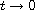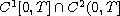Electron. J. Differential Equations, Vol. 2016 (2016), No. 273, pp. 1-8.

### Solvability of singular second-order initial-value problems Petio Kelevedjiev

Abstract:
This article concerns the solvability of the initial-value problem x''=f(t,x,x'), x(0)=A, x'(0)=B, where the scalar function f may be unbounded as. Using barrier strip type arguments, we establish the existence of monotone and/or positive solutions in.

Submitted April 8, 2016. Published October 12, 2016.
Math Subject Classifications: 34A12, 34A36.
Key Words: Initial value problem; second order differential equation; singularity; existence; barrier conditions.

Show me the PDF file (194 KB), TEX file for this article.Petio Kelevedjiev Technical University of Sofia Branch Sliven, Bulgaria email: keleved@mailcity.com